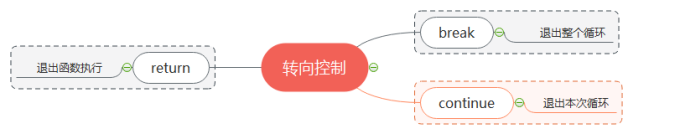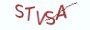Python基础教程

# 7.3.1 理解转向控制

`条件控制是指根据表达式的值，来有条件地执行程序中的指令。循环控制是指让程序指令连续反复地执行`# 7.3.2 break-退出整个循环

break有打破和中途休息的意思，打破循环，意味着终止整个循环。通俗地进行解释，break是循环的终结者，类似T-800机器人一样的存在。

```sum_of_numbers = 0
for number in range(1000):
sum_of_numbers = sum_of_numbers + number
if sum_of_numbers > 2020:
# 如果sum_of_numbers指向的值大于2020，就退出循环
break```

```(1) 在循环中使用条件控制判断变量sum_of_numbers指向的值
(2) 如果该值大于2020，就执行break退出循环```

```sum_of_numbers = 0
for number in range(2,  100):
for divisor in range(2,  number):
if number % divisor == 0:
# %是求余运算符，如果当前number与_的余数为0
# 说明能为其它数整除，则肯定不是质数，执行break退出循环
break
else:
# 循环正常退出，说明当前number为质数
sum_of_numbers = sum_of_numbers + number```

```(1) 在内层for循环中，当number为其它自然数整除时就退出当前的循环。
(2) 如果内层的循环系正常退出，说明不能被其它自然数整除，那么它是质数。
(3) 退出内层的for循环，不会对外层的for循环造成影响。```

# 7.3.3 continue-退出本次循环

```sum_of_numbers = 0
for number in range(1000):
if number % 2 == 0:
# % 是Python中的求余运算符，如果与2相余后的余数为0
# 说明当前number是偶数，那么退出本次循环
continue

# 显然地，如果number为奇数
# 就会运行下面的代码
# sum_of_numbers存储的是所有奇数的和
sum_of_numbers = sum_of_numbers + number

else:
print(sum_of_numbers)```

```(1) %是Python中的求余计算符，如果数与2相余后的余数为0，说明是偶数。

(2) 如果不为偶数，那么就会继续执行循环体中的代码，计算所有奇数的和。```

```"""

* * *
* *
*
"""
# 定义变量triangle，用来存储打印的字符串
# 在Python中用英文引号括住的都为字符串
# 可以使用+符号对字符串进行拼接
# 在字符串中使用\n符号可以断行
triangle=""
# 定义变量rows表示行数
rows = 3
# 定义cols变量表示列数
cols  = 5

for row in range(rows):
# 定义is_even_number变量，判断当前行是否为偶数
is_even_number = 1 if row % 2 == 0 else 0
# 定义started变量，判断是否开始打印*符号
started = 0

for col in range(cols):
# 如果当前列的编号等于当前行的编号，就开始打印*符号
if col == row:
triangle = triangle+"*"
started = 1
continue

# 如果已经开始打印*符号，且列的编号在正确范围以内
if started and col<=cols-row:
if is_even_number:
triangle = triangle + "*" if col % 2 == 0 else triangle + " "
else:
triangle = triangle + "*" if col % 2 != 0 else  triangle + " "
else:
triangle = triangle + " "

# 在字符串中拼接\n符号，可以进行断行
triangle = triangle+"\n"
else:
print(triangle)```

```* * *
* *
*```

(1) 根据图形本身的特征，去寻找规律

(2) 很显然倒三角形中一共三行五列，使用*符号与空格符号来共同输出三角形

(3) 当列的编号与本次循环中的行的编号相等时，开始打印*符号，这个从图形本身的特征可以发现这个规律。

(4) 再者，倒三角形中每一层的*数目越来越少且居中，打印*的有效范围在列数减去当前行编号的范围之内。在Python中，break与continue都只能用于循环结构。在循环中使用了break,意味着非正常退出，不会执行else中的代码。

# 7.3.4 return-退出函数执行

` 对于break,continue，return等关键字的定义和用法，同学们同样可以在交互模式中进行查找， 不再赘述。`

# 7.3.5 知识要点

`(1) 转向控制一般用于循环结构和函数中，用来控制循环和函数的执行过程。 (2) Python通过break,continue,return来实现转向控制。 (3) break是终止整个循环，continue是终止本次循环 (4) return用于退出函数的执行，通常会返回一个值给调用方。`

# 7.3.6 最具实力的小班培训

`(1) Python后端工程师高薪就业班，月薪11K-18K，免费领取课程大纲(2) Python爬虫工程师高薪就业班，年薪十五万，免费领取课程大纲(3) Java后端开发工程师高薪就业班，月薪11K-20K, 免费领取课程大纲(4) Python大数据分析，量化投资就业班，月薪12K-25K,免费领取课程大纲`### 已有4位薯条发表了看法：

•访客  评论于 2020-05-15 20:21:28  回复

老师，”triangle = triangle+"\n"else:“ 这句的”else：”是什么意思啊

•访客  评论于 2020-05-16 06:50:48  回复

这是条件控制语句中的三元运算符，你需要复习下条件控制这一节

•访客  评论于 2020-09-11 16:07:25  回复

计算质数代码的注释写错了吧,“# %是求余运算符，如果当前number与2的余数为0 ”应该是number与_的余数为0

•薯条老师  评论于 2020-10-14 08:59:09  回复

是写错了，感谢指正，已修改

## 注册账号